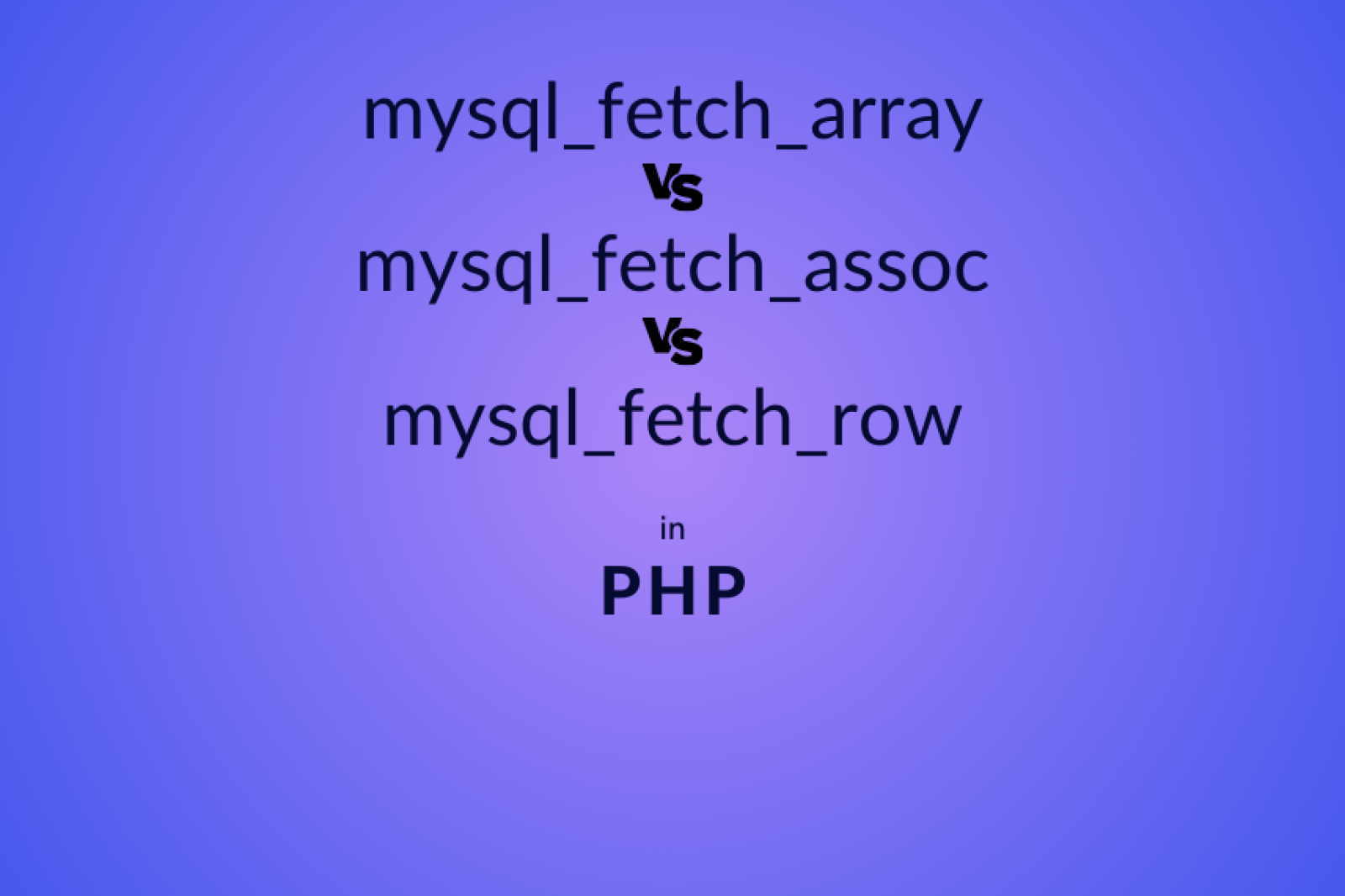## Understanding the Differences Between mysql_fetch_array(), mysql_fetch_assoc(), and mysql_fetch_row() in PHP

Many of the PHP newbies get confused with `mysql_fetch_row()`, `mysql_fetch_object()`, `mysql_fetch_assoc()`, `mysql_fetch_array()` functions. In this post we will check then in detail with example.

First of all, create a table called `users` by running the below query:

``````create table users(
id INT NOT NULL AUTO_INCREMENT,
email VARCHAR(250) NOT NULL,
PRIMARY KEY ( id )
);``````

Insert some testing data as well:

`````` INSERT INTO `users` (`id`, `username`, `email`) VALUES (NULL, 'Piers', 'piers@test.com');
INSERT INTO `users` (`id`, `username`, `email`) VALUES (NULL, 'Warren', 'warren@test.com');
INSERT INTO `users` (`id`, `username`, `email`) VALUES (NULL, 'Tim', 'tim@test.com');
INSERT INTO `users` (`id`, `username`, `email`) VALUES (NULL, 'Joseph', 'joseph@test.com');
INSERT INTO `users` (`id`, `username`, `email`) VALUES (NULL, 'Robert', 'robert@test.com');
INSERT INTO `users` (`id`, `username`, `email`) VALUES (NULL, 'Peter', 'peter@test.com');
INSERT INTO `users` (`id`, `username`, `email`) VALUES (NULL, 'Boris', 'boris@test.com');``````

Create a file called `index.php` and try to use the below code for these functions.

### mysqli_fetch_row()

Fetch a result row as a numeric way. This function will return a row where the values will come in the order as they are defined in the SQL query, and the keys will span from 0 to one less than the number of columns selected.

``````<?php
//connect database

//select values from users table
\$sql    = "select * from users where email='tim@test.com'";
\$result = mysqli_query(\$con,\$sql);
\$row    = mysqli_fetch_row(\$result);

// access individual values
echo \$userid    = \$row;
echo \$useremail = \$row;

mysqli_close(\$con);``````

Output:

``````3
Tim
tim@test.com``````

Herewith this  `mysqli_fetch_row()` function, if you are fetching the selected records then their order matters while getting the result.

For example, if you have written, `"select id, username, email from users"` then,

`\$rows;` will have the value of the id
`\$rows;` will have the value of username
`\$rows;` will have the value of the email

but if you write, `"select id, email, username from users"`  then,

`\$rows;` will have the value of the id
`\$rows;` will have the value of the email
`\$rows;` will have the value of username

### mysqli_fetch_assoc()

Fetch a result row as an associative array. This function will return a row as an associative array where the column names will be the keys storing corresponding value.

``````<?php

//connect database

//select values from users table
\$sql    = "select * from users where email='tim@test.com'";
\$result = mysqli_query(\$con,\$sql);
\$row    = mysqli_fetch_assoc(\$result);

// access individual values
echo \$userid    = \$row['id'];
echo \$useremail = \$row['email'];

mysqli_close(\$con);``````

Output:

``````3
Tim
tim@test.com``````

### mysqli_fetch_array()

Fetch a result row as an associative array & numeric array both. It returns an array with both the contents of `mysqli_fetch_row()` and `mysqli_fetch_assoc()` merged into one. It will have both numeric and string keys.

``````<?php

//connect database

//select values from users table
\$sql    = "select * from users where email='tim@test.com'";
\$result = mysqli_query(\$con,\$sql);
\$row    = mysqli_fetch_array(\$result);

print_r(\$row);

mysqli_close(\$con);``````

Output:

``````Array
(
 => 3
[id] => 3
 => Tim
 => tim@test.com
[email] => tim@test.com
)``````

Access individual values by string keys:

``````echo \$userid    = \$row['id'];
echo \$useremail = \$row['email'];``````

Output:

``````3
Tim
tim@test.com``````

Access individual values by numeric keys:

``````echo \$userid    = \$row;
echo \$useremail = \$row;``````

Output:

``````3
Tim
tim@test.com``````

### mysqli_fetch_object()

Fetch a result row as an object.

``````<?php

//connect database

//select values from users table
\$sql    = "select * from users where email='tim@test.com'";
\$result = mysqli_query(\$con,\$sql);
\$row    = mysqli_fetch_object(\$result);

// access individual values as object
echo \$userid    = \$row->id;
echo \$useremail = \$row->email;

mysqli_close(\$con);``````

Output:

``````3
Tim
tim@test.com``````

Now you should have clear understanding of these functions.#### Ketan Patel

As a backend and ecommerce developer, I have extensive experience in implementing robust and scalable solutions for ecommerce websites and applications. I have a deep understanding of server-side technologies and have worked with various programming languages. I have experience in integrating with payment gateways, and other third-party services.